• 使用Matlab运行程序的时候，我们经常需要知道或比较不同程序的具体运行时间，其程序代码如下： st=cputime; % 程序开始运行时的cpu时间 % 程序开始 …… % 程序结束 et=cputime-st % 计算程序运行所用的...
 使用Matlab运行程序的时候，我们经常需要知道或比较不同程序的具体运行时间，其程序代码如下：
st=cputime;        % 程序开始运行时的cpu时间
% 程序开始
……
% 程序结束
et=cputime-st        % 计算程序运行所用的时间
经常我们需要计算我们程序到底运行多长时间，这样可以比较程序的执行效率。当然这个对于只有几秒钟的小程序没有什么意义，但是对于大程序就有很重要的意义了。
下面我们就说说Matlab中计算程序运行时间的三种常用方法吧！
注意：三种方法由于使用原理不一样，得到结果可能有一定的差距！
1、tic和toc组合(使用最多的)
计算tic和toc之间那段程序之间的运行时间，它的经典格式为
tic
。。。。。。。。。。
toc
复制代码
换句话说程序，程序遇到tic时Matlab自动开始计时，运行到toc时自动计算此时与最近一次tic之间的时间。这个有点拗口，下面我们举个例子说明
% by dynamic of Matlab技术论坛
% see also http://www.matlabsky.com
% contact me matlabsky@gmail.com
% 2009-08-18 12:08:47
clc
tic;%tic1
t1=clock;
for i=1:3
tic ;%tic2
t2=clock;
pause(3*rand)
%计算到上一次遇到tic的时间，换句话说就是每次循环的时间
disp(['toc计算第',num2str(i),'次循环运行时间：',num2str(toc)]);
%计算每次循环的时间
disp(['etime计算第',num2str(i),'次循环运行时间：',num2str(etime(clock,t2))]);
%计算程序总共的运行时间
disp(['etime计算程序从开始到现在运行的时间:',num2str(etime(clock,t1))]);
disp('======================================')
end
%计算此时到tic2的时间，由于最后一次遇到tic是在for循环的i=3时，所以计算的是最后一次循环的时间
disp(['toc计算最后一次循环运行时间',num2str(toc)])
disp(['etime程序总运行时间：',num2str(etime(clock,t1))]);
复制代码
运行结果如下，大家可以自己分析下
toc计算第1次循环运行时间：2.5628
etime计算第1次循环运行时间：2.562
etime计算程序从开始到现在运行的时间:2.562
======================================
toc计算第2次循环运行时间：2.8108
etime计算第2次循环运行时间：2.813
etime计算程序从开始到现在运行的时间:5.375
======================================
toc计算第3次循环运行时间：2.0462
etime计算第3次循环运行时间：2.046
etime计算程序从开始到现在运行的时间:7.421
======================================
toc计算最后一次循环运行时间2.0479
etime程序总运行时间：7.421
复制代码
2、etime(t1,t2)并和clock配合
来计算t1，t2之间的时间差，它是通过调用windows系统的时钟进行时间差计算得到运行时间的，应用的形式
t1=clock;
。。。。。。。。。。。
t2=clock;
etime(t2,t1)
复制代码
至于例子我就不举了，因为在上面的例子中使用了etime函数了
3、cputime函数来完成
使用方法和etime相似，只是这个是使用cpu的主频计算的，和前面原理不同，使用格式如下
t0=cputime
。。。。。。。。。。。。。
t1=cputime-t0
复制代码
上面说到了三种方法，都是可以进行程序运行时间计算的，但是Matlab官方推荐使用tic/toc组合，When timing the duration of an event, use the tic and toc functions instead of clock or etime.
至于大家可以根据自己的喜好自己选择，但是使用tic/toc的时候一定要注意，toc计算的是与最后一次运行的tic之间的时间，不是第一个tic，更不是第二个。。。。。

展开全文• 综述 评价程序的运行时间是一项十分重要的工作。matlab中使用tic+toc可以完成。程序遇到tic开始计时，运行到toc时...若想将用运行时间记录下来，则这样使用toc tic %xxxx %ur code mytimer1=toc; disp(mytimer1) ...
综述
评价程序的运行时间是一项十分重要的工作。matlab中使用tic+toc可以完成。程序遇到tic开始计时，运行到toc时计算此时与最近一次tic间的时间。时间单位是s。
代码
%test脚本。若想将用运行时间记录下来，则这样使用toc
tic
%xxxx
%ur code
mytimer1=toc;
disp(mytimer1)



展开全文• 比如DTW最常用的地方，语音识别中，同一个字母，由不同人发音，长短肯定不一样，把声音记录下来以后，它的信号肯定是很相似的，只是在时间上不太对整齐而已。所以我们需要用一个函数拉长或者缩短其中一个信号，使得...
动态时间规整算法，

就是把两个代表同一个类型的事物的不同长度序列进行时间上的“对齐”。比如DTW最常用的地方，语音识别中，同一个字母，由不同人发音，长短肯定不一样，把声音记录下来以后，它的信号肯定是很相似的，只是在时间上不太对整齐而已。所以我们需要用一个函数拉长或者缩短其中一个信号，使得它们之间的误差达到最小。

在时间序列中，需要比较相似性的两段时间序列的长度可能并不相等，在语音识别领域表现为不同人的语速不同。而且同一个单词内的不同音素的发音速度也不同，比如有的人会把“A”这个音拖得很长，或者把“i”发的很短。另外，不同时间序列可能仅仅存在时间轴上的位移，亦即在还原位移的情况下，两个时间序列是一致的。在这些复杂情况下，使用传统的欧几里得距离无法有效地求的两个时间序列之间的距离（或者相似性）。

DTW通过把时间序列进行延伸和缩短，来计算两个时间序列性之间的相似性：如上图所示，上下两条实线代表两个时间序列，时间序列之间的虚线代表两个时间序列之间的相似的点。DTW使用所有这些相似点之间的距离的和，称之为归整路径距离(Warp Path Distance)来衡量两个时间序列之间的相似性。

再来看看运动捕捉，比如当前有一个很快的拳击动作，另外有一个未加标签的动作，我想判断它是不是拳击动作，那么就要计算这个未加标签的动作和已知的拳击动作的相似度。但是呢，他们两个的动作长度不一样，比如一个是100帧，一个是200帧，那么这样直接对每一帧进行对比，计算到后面，误差肯定很大，那么我们把已知拳击动作的每一帧找到无标签的动作的对应帧中，使得它们的距离最短。这样便可以计算出两个运动的相似度，然后设定一个阈值，满足阈值范围就把未知动作加上“拳击”标签。

欧式距离的计算方法：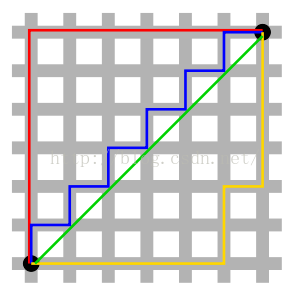图来自维基百科，其中红黄蓝线均为曼哈顿距离，绿色为欧式距离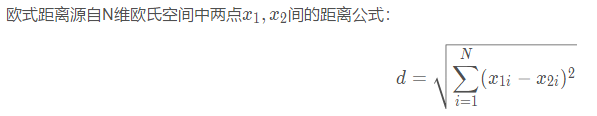DTW的具体计算过程：

下面我们来总结一下DTW动态时间规整算法的简单的步骤：

1. 首先肯定是已知两个或者多个序列，但是都是两个两个的比较，所以我们假设有两个序列A={a1,a2,a3,...,am}  B={b1,b2,b3,....,bn}，维度m>n

2. 然后用欧式距离计算出每序列的每两点之间的距离，D(ai,bj) 其中1≤i≤m，1≤j≤n

画出下表：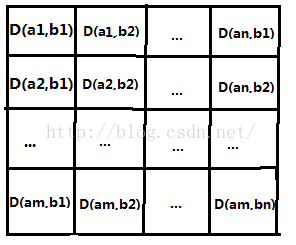3.  接下来就是根据上图将最短路径找出来。从D(a1,a2)沿着某条路径到达D(am,bn)。找路径满足：假如当前节点是D(ai,bj)，那么下一个节点必须是在D(i+1,j)，D(i,j+1)，D(i+1,j+1)之间选择，并且路径必须是最短的。计算的时候是按照动态规划的思想计算，也就是说在计算到达第(i,j)个节点的最短路径时候，考虑的是左上角也即第(i-1,j)、(i-1,j-1)、(i,j-1)这三个点到(i,j)的最短距离。

4. 接下来按照回溯法输出路径，从D(a1,b1)到D(am,bn)。他们的总和就是就是所需要的DTW距离

举个栗子：

已知：两个列向量a=[8 9 1]'，b=[ 2 5 4 6]'，其中'代表转置，也就是把行向量转换为列向量了

求：两个向量利用动态时间规整以后的最短距离

第一步：计算对应点的欧式距离矩阵d【注意开根号】

6
3
4
2
7
4
5
3
1
4
3
5

第二步：计算累加距离D【从6出发到达5的累加距离】

6
9
13
15
13
10
14
16
14
14
13
18
计算方法如下：
D(1,1)=d(1,1)=6

D(1,2)=D(1,1)+d(1,2)=9

...

D(2,2)=min(D(1,2),D(1,1),D(2,1))+d(2,2)=6+4=10

...

D(m,n)=min(D(m-1,n),D(m-1,n-1),D(m,n-1))+d(m,n)

matlab的代码如下：

dtw.m

function [Dist,D,k,w,rw,tw]=dtw(r,t,pflag)
%
% [Dist,D,k,w,rw,tw]=dtw(r,t,pflag)
%
% Dynamic Time Warping Algorithm
% Dist is unnormalized distance between t and r
% D is the accumulated distance matrix
% k is the normalizing factor
% w is the optimal path
% t is the vector you are testing against
% r is the vector you are testing
% rw is the warped r vector
% tw is the warped t vector
% pflag  plot flag: 1 (yes), 0(no)
%
% Version comments:
% rw, tw and pflag added by Pau Mic

[row,M]=size(r); if (row > M) M=row; r=r'; end;
[row,N]=size(t); if (row > N) N=row; t=t'; end;
d=sqrt((repmat(r',1,N)-repmat(t,M,1)).^2); %this makes clear the above instruction Thanks Pau Mic
d
D=zeros(size(d));
D(1,1)=d(1,1);

for m=2:M
D(m,1)=d(m,1)+D(m-1,1);
end
for n=2:N
D(1,n)=d(1,n)+D(1,n-1);
end
for m=2:M
for n=2:N
D(m,n)=d(m,n)+min(D(m-1,n),min(D(m-1,n-1),D(m,n-1))); % this double MIn construction improves in 10-fold the Speed-up. Thanks Sven Mensing
end
end

Dist=D(M,N);
n=N;
m=M;
k=1;
w=[M N];
while ((n+m)~=2)
if (n-1)==0
m=m-1;
elseif (m-1)==0
n=n-1;
else
[values,number]=min([D(m-1,n),D(m,n-1),D(m-1,n-1)]);
switch number
case 1
m=m-1;
case 2
n=n-1;
case 3
m=m-1;
n=n-1;
end
end
k=k+1;
w=[m n; w]; % this replace the above sentence. Thanks Pau Mic
end

% warped waves
rw=r(w(:,1));
tw=t(w(:,2));

%%%%%%%%%%%%%%%%%%%%%%%%%%%%%%%%%%%%%%%%%%%%%%%%%%%%%%%%%%%%%%%%%%%%%%%%%%%
if pflag

% --- Accumulated distance matrix and optimal path
figure('Name','DTW - Accumulated distance matrix and optimal path', 'NumberTitle','off');

main1=subplot('position',[0.19 0.19 0.67 0.79]);
image(D);
cmap = contrast(D);
colormap(cmap); % 'copper' 'bone', 'gray' imagesc(D);
hold on;
x=w(:,1); y=w(:,2);
ind=find(x==1); x(ind)=1+0.2;
ind=find(x==M); x(ind)=M-0.2;
ind=find(y==1); y(ind)=1+0.2;
ind=find(y==N); y(ind)=N-0.2;
plot(y,x,'-w', 'LineWidth',1);
hold off;
axis([1 N 1 M]);
set(main1, 'FontSize',7, 'XTickLabel','', 'YTickLabel','');

colorb1=subplot('position',[0.88 0.19 0.05 0.79]);
nticks=8;
ticks=floor(1:(size(cmap,1)-1)/(nticks-1):size(cmap,1));
mx=max(max(D));
mn=min(min(D));
ticklabels=floor(mn:(mx-mn)/(nticks-1):mx);
colorbar(colorb1);
set(colorb1, 'FontSize',7, 'YTick',ticks, 'YTickLabel',ticklabels);
set(get(colorb1,'YLabel'), 'String','Distance', 'Rotation',-90, 'FontSize',7, 'VerticalAlignment','bottom');

left1=subplot('position',[0.07 0.19 0.10 0.79]);
plot(r,M:-1:1,'-b');
set(left1, 'YTick',mod(M,10):10:M, 'YTickLabel',10*rem(M,10):-10:0)
axis([min(r) 1.1*max(r) 1 M]);
set(left1, 'FontSize',7);
set(get(left1,'YLabel'), 'String','Samples', 'FontSize',7, 'Rotation',-90, 'VerticalAlignment','cap');
set(get(left1,'XLabel'), 'String','Amp', 'FontSize',6, 'VerticalAlignment','cap');

bottom1=subplot('position',[0.19 0.07 0.67 0.10]);
plot(t,'-r');
axis([1 N min(t) 1.1*max(t)]);
set(bottom1, 'FontSize',7, 'YAxisLocation','right');
set(get(bottom1,'XLabel'), 'String','Samples', 'FontSize',7, 'VerticalAlignment','middle');
set(get(bottom1,'YLabel'), 'String','Amp', 'Rotation',-90, 'FontSize',6, 'VerticalAlignment','bottom');

% --- Warped signals
figure('Name','DTW - warped signals', 'NumberTitle','off');

subplot(1,2,1);
set(gca, 'FontSize',7);
hold on;
plot(r,'-bx');
plot(t,':r.');
hold off;
axis([1 max(M,N) min(min(r),min(t)) 1.1*max(max(r),max(t))]);
grid;
legend('signal 1','signal 2');
title('Original signals');
xlabel('Samples');
ylabel('Amplitude');

subplot(1,2,2);
set(gca, 'FontSize',7);
hold on;
plot(rw,'-bx');
plot(tw,':r.');
hold off;
axis([1 k min(min([rw; tw])) 1.1*max(max([rw; tw]))]);
grid;
legend('signal 1','signal 2');
title('Warped signals');
xlabel('Samples');
ylabel('Amplitude');

end下面是测试代码

test.m

clear
clc
a=[8 9 1 9 6 1 3 5]';
b=[2 5 4 6 7 8 3 7 7 2]';
[Dist,D,k,w,rw,tw] = DTW(a,b,1);
fprintf('最短距离为%d\n',Dist)
fprintf('最优路径为')
w

测试结果：

d =

6     3     4     2     1     0     5     1     1     6
7     4     5     3     2     1     6     2     2     7
1     4     3     5     6     7     2     6     6     1
7     4     5     3     2     1     6     2     2     7
4     1     2     0     1     2     3     1     1     4
1     4     3     5     6     7     2     6     6     1
1     2     1     3     4     5     0     4     4     1
3     0     1     1     2     3     2     2     2     3

最短距离为27
最优路径为
w =

1     1
1     2
1     3
1     4
1     5
1     6
2     6
3     7
4     8
5     9
6    10
7    10
8    10规整以后的可视化结果如下：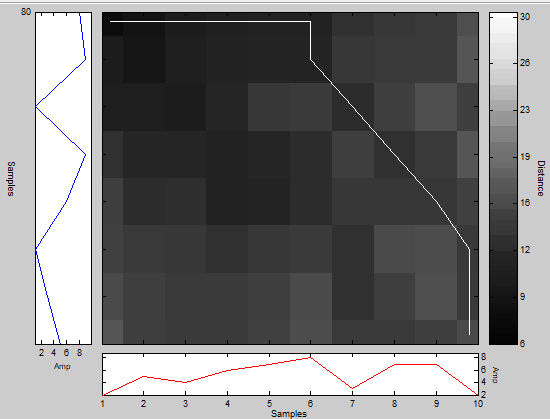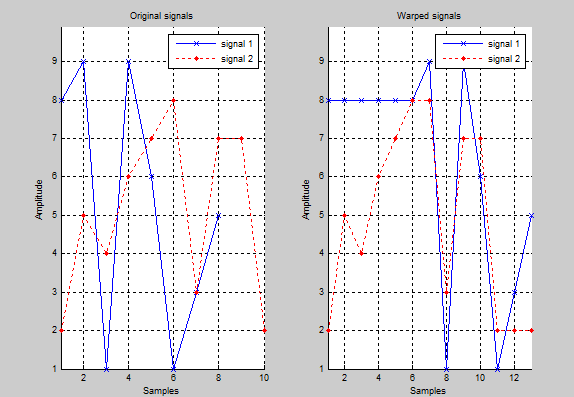---------------------
作者：风翼冰舟
来源：CSDN
原文：https://blog.csdn.net/zb1165048017/article/details/49226315
版权声明：本文为博主原创文章，转载请附上博文链接！
展开全文• Tic和toc函数可以计算运行一段时间代码。 例如： 1 2 3 4 5 6 7 8 9 10 11 12 13 14 15 16 17 18 19 20 21 22 23 24 25 clc tic d=zeros(1,10000); fori=1:10000 d(i)=i; end toc...


Tic和toc函数可以计算运行一段时间的代码。
例如：

1
2
3
4
5
6
7
8
9
10
11
12
13
14
15
16
17
18
19
20
21
22
23
24
25

clc

tic

d=zeros(1,10000);

for i=1:10000

    d(i)=i;

end

toc

tic

c=1;

for i=1:10000

    c=[c:i];

end

toc

运行结果如下：

1
2
3

Elapsed time is 0.000158 seconds.

Elapsed time is 0.152307 seconds.

只要用tic和toc函数，不需要自己计算前后时间的差，tic函数会记录起始时刻，toc函数会自动计算时间差。
通过这个程序，可以发现，先把矩阵的大小确定再给矩阵的赋值的方法比边赋值边改变矩阵维数的方法更节省时间。

1
2
3
4
5
6
7
8
9
10
11
12
13
14
15
16
17
18
19
20
21
22
23
24
25
26
27
28
29
30
31
32
33

%本程序用来比较两种计算恰定方程的时间和精确度

%第一种使用矩阵求逆的方法

%第二种使用矩阵的除法

clc;

a=rand(100)+1.e10;

x=ones(100,1);

b=a*x;

tic

y=inv(a)*b;

toc

err=norm(y-x)          %结果与精确解的范2误差

res=norm(a*y-b)        %方程的范2误差

tic

y=a\b;

toc

err=norm(y-x)

res=norm(a*y-b)

本文转自二郎三郎博客园博客，原文链接：http://www.cnblogs.com/haore147/p/3641434.html，如需转载请自行联系原作者


展开全文• ## Matlab 计时函数

千次阅读 2017-03-16 16:45:16
当你需要计算一组Matlab操作的运行时间时，可以使用tic和toc函数。tic函数启动一个秒表，表示计时开始；toc则停止这个秒表，表示计时结束，并计算出所经历的时间（单位为秒）。比如，下面的代码连续两次计算plot...
• 直接把建模作业贴上来了，代码是参考一个学长写的，图解的话百度文库里有好多不错的资料，配合这个代码应该...%用于记录运行时间 X=[114 50 99 30 60 26 29 7 53 40 102 3 32 12 100 38 5 2 91 5 46 105 95 94 34 116算法 注释
• MATLAB（R2017a）中运行该模型后，MATLAB工作空间中会有类型为Dataset的logsout变量，下面是读取并显示其中数据的MATLAB代码： % 读取Simulink.SimulationData.Dataset中的数据 data = logsout.get(1).Values.Data...
• 本文是《科学计算与MATLAB语言》专题七第1小节的学习笔记，如果大家有时间的话，可以去听听课，...从符号对象的建立、运算到符号矩阵的运算，本文都有详细的记录，每个代码我都跑过一遍，可以直接复制到MATLAB运行数学建模
• 简单地说，tic和toc是用来记录matlab命令执行的时间 tic用来保存当前时间，而后使用toc来记录...Tic和toc函数可以计算运行一段时间代码 例如： clc tic d=zeros(1,10000); for i=1:10...
• 我们知道 matlab 中可以使用，tic 和 toc 命令记录代码运行时间。 tic disp('hello,world') toc 如果需要自定义输出时间的形式可以这样 tic disp('hello,world') t = toc; disp(['耗时 ',num2str(t),' s'...
• 此外，它提供各种设置以满足不同的分析需求，包括（1）处理单个数据记录，（2）批处理多个数据文件，（3）滑动窗口计算，（4）调用，（5）显示中间数据和最终结果，（6）调整输入参数，以及（7）在运行后或在分析...
• 压缩包里有一个内含1000个24位十六进制数的txt文件，假定这1000个数据为当前的RFID标签，本程序实现了从文件读取数据，转换格式后进行识别，并转换为原格式后按识别顺序输出到txt文档中，附带了记录程序运行时间的...
• 我计划把我的实现过程记录下来，而不是只是呈现一个最终的系统界面给大家。我每天添加一些类容，公布一些进展，并且上传不同阶段的程序。这样做的好处是可以把整个系统分散开来，不同会员可以根据自己的需要摘取系统...
• 我计划把我的实现过程记录下来，而不是只是呈现一个最终的系统界面给大家。我每天添加一些类容，公布一些进展，并且上传不同阶段的程序。这样做的好处是可以把整个系统分散开来，不同会员可以根据自己的需要摘取系统...
• 我计划把我的实现过程记录下来，而不是只是呈现一个最终的系统界面给大家。我每天添加一些类容，公布一些进展，并且上传不同阶段的程序。这样做的好处是可以把整个系统分散开来，不同会员可以根据自己的需要摘取系统...
• 我计划把我的实现过程记录下来，而不是只是呈现一个最终的系统界面给大家。我每天添加一些类容，公布一些进展，并且上传不同阶段的程序。这样做的好处是可以把整个系统分散开来，不同会员可以根据自己的需要摘取系统...
• 我计划把我的实现过程记录下来，而不是只是呈现一个最终的系统界面给大家。我每天添加一些类容，公布一些进展，并且上传不同阶段的程序。这样做的好处是可以把整个系统分散开来，不同会员可以根据自己的需要摘取系统...
• 我计划把我的实现过程记录下来，而不是只是呈现一个最终的系统界面给大家。我每天添加一些类容，公布一些进展，并且上传不同阶段的程序。这样做的好处是可以把整个系统分散开来，不同会员可以根据自己的需要摘取系统...
• python和matlab都有非常友好的记录时间的方式，且不说python的time，datetime工具包，matlab的tic，toc命令简单好记，都是程序时间很好的记录工具，可以帮助后续代码的持续优化和改善。但是shell脚本如何记录运行了...shell
• 争取明天结束前把论文模型的matlab代码全码出来，已经码了两百多行了。 已经过去三周了。 第一周，matlab新手上路，初识matlab语言，初步掌握简单的模型制作； 第二周+第三周两三天，设计好论文模型； 第三周，...
• 信噪比计算优化过程记录（第一份C++代码的优化记录） 工作内容介绍：SNR计算Matlab代码转C++ ...Matlab 代码运行时间为：13.463308s 第一版C++运行时间为：14375 ms 优化过程： 优化1：弃用pu...
• 2>自己编写回测模块，这个比较简单，具体方式是，在原有逻辑基础上，一条条读取历史行情记录（例如.txt,xls,csv文件，淘宝有卖，也可以用本库的TICK数据收集例子收集），回测完毕，再加载到其它第三方Python图形库...
• 刚开始学习利用Simulink自动生成可以在STM32单片机运行代码，摸索的过程中遇到了很多挫折，希望以这种方式来记录自己的成长与进步，以下的四个案例花了将近4天的时间，经过亲自测试均可正常运行 配置环境：MATLAB...快速原型 CubeMX
• Matlab鼠标画图,用pop-up menu选择画图标记,颜色edit记录鼠标坐标 但是功能比较简单，只能画线，不能画矩形、椭圆等 lyqmath兄的也提出了这个问题。 为此花了一天的时间好好研究了一下，尝试了很多方法，当前这种...
• Matlab鼠标画图,用pop-up menu选择画图标记,颜色edit记录鼠标坐标 但是功能比较简单，只能画线，不能画矩形、椭圆等 lyqmath兄的也提出了这个问题。 为此花了一天的时间好好研究了一下，尝试了很多方法，当前这种...
• Matlab鼠标画图,用pop-up menu选择画图标记,颜色edit记录鼠标坐标 但是功能比较简单，只能画线，不能画矩形、椭圆等 lyqmath兄的也提出了这个问题。 为此花了一天的时间好好研究了一下，尝试了很多方法，当前这种...
• 支持 MDF NDF文件修复 支持备份文件修复完美支持各种字符集，支持从MDF文件反删除记录。 支持 SQL数据库各种字段类型 支持压缩 立即下载 1积分/C币时间：2018-06-12 doc文件 管家婆辉煌版7.x数据恢复(出现因病毒或...# matlab记录代码运行时间matlab 订阅